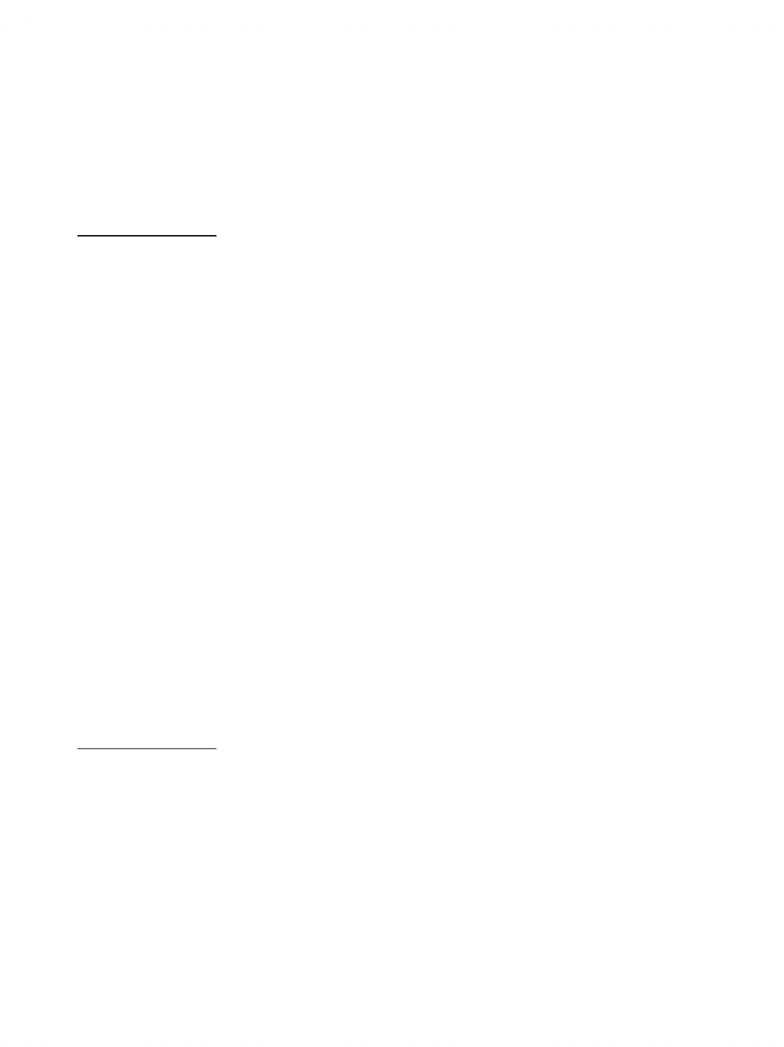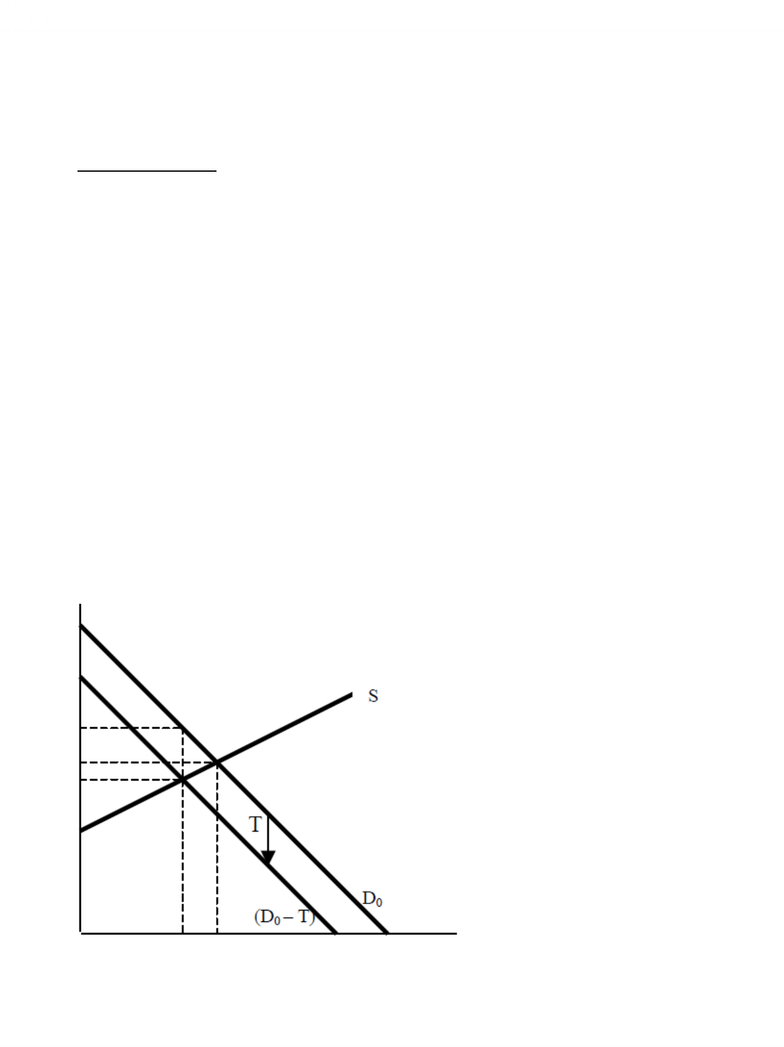Study Guides (380,000)
CA (150,000)
UTSC (10,000)
MGEA02H3 (100)
all (20)

# MGEA02H3 Study Guide - Economic Surplus, Swordfish, Deadweight Loss

Department
Economics for Management Studies
Course Code
MGEA02H3
Professor
all

This preview shows pages 1-2. to view the full 6 pages of the document.Demand, Supply and Equilibrium
1. Movement and Shift of Supply/Demand Curve
Knowledge Summary:
Movement along the supply/demand curve:
- Relevant factor: Price
- When price change, the quantity supplied/consumed will change, thus there will be a movement along
the supply/demand curve
Demand is usually downward sloping and Supply upward sloping
Intersection of Demand and Supply curves is the equilibrium point
Change in quantity demanded/quantity supplied are movements along the demand/supply curve and
change in the demand/supply is a shift of the demand/supply curve
The Demand/Supply Curve is the sum of Individual demand/supply of the product
Factors that Shift Demand:
1. Taxes/Subsidies.
2. Change in Prices of related goods (Substitutes/Complementary Goods)
3. Change in Income
4. Change in Preferences
Factors That Shift Supply:
1. Prices of Inputs (as prices rise, Supply falls)
2. Number of Firms (as firms rise, Supply Rises)
3. Technology (a new breakthrough increases supply)
4. Taxes/Subsidies
Questions to be asked:
If there is a change in situation, what would be a possible impact on equilibrium price and quantity?
- Determine the original equilibrium
- Figure out the factor that changed in the situation
- Shift the demand/supply curve to the relevant direction
- Determine the new equilibrium
- Compare the old and new equilibrium; determine the change in price and quantity

Only pages 1-2 are available for preview. Some parts have been intentionally blurred.2. Supply and Demand function
Knowledge Summary:
1. Demand function: the relationship between the quantities the consumers would like to consume for a
particular price
2. Supply function: the relationship between the quantities the suppliers are willing to produce for a
particular price
3. Equilibrium point: the intersection of demand curve and supply curve
- Qd = Qs
4. Consumer surplus
- Graphically, it is the area between the Y- axis, demand curve and horizontal line for P = P* (equilibrium
price)
- Theoretically, it is the excess of total consumer benefits over total expenditure
5. Producer surplus
- Graphically, it is the area between the Y axis, supply curve and horizontal line for P = P* (equilibrium
price)
- Theoretically, it is the excess of total revenue over total variable cost
6. Tax imposed by the government
- If the tax is levied on the consumers, demand curve will shift down by T
- If the tax is levied on the suppliers, supply curve will shift up by T
- No matter the tax is levied on consumers or suppliers, the share of taxes between consumers and
suppliers will be the same
###### You're Reading a Preview

Unlock to view full version